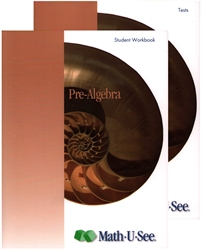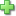# Math-U-See Pre-Algebra - Student Pack

Publisher: Math-U-See
Hardcover
Price: \$48.00

See series description for full review.

Pre-Algebra teaches basic rules of solving equations using the associative, commutative, and other properties. Simple operations with polynomials are demonstrated with place-value blocks for conceptual understanding before being taught conventionally. Geometry concepts include surface area, volume, and the Pythagorean theorem.

Scope and Sequence:

• Lesson 01: Negative Numbers: Addition
• Lesson 02: Negative Numbers: Subtraction
• Lesson 03: Negative Numbers: Multiplication
• Lesson 04: Negative Numbers: Division
• Lesson 05: Exponents
• Lesson 06: Place Value with Expanded and Exponential Notation
• Lesson 07: Negative Numbers with Exponents
• Lesson 08: Roots and Radicals
• Lesson 09: Solve for an Unknown with Additive Inverse
• Lesson 10: Pythagorean Theorem
• Lesson 11: Associative and Commutative Properties
• Lesson 12: Distributive Property
• Lesson 13: Solve for an Unknown with Multiplicative Inverse
• Lesson 14: Solve for an Unknown with Order of Operations
• Lesson 15: Surface Area of Solids
• Lesson 16: Convert Celsius to Fahrenheit
• Lesson 17: Convert Fahrenheit to Celsius
• Lesson 18: Absolute Value
• Lesson 19: Ratio and Proportion
• Lesson 20: Similar Polygons
• Lesson 21: Least Common Multiple and Prime Factorization
• Lesson 22: Greatest Common Factor
• Lesson 24: Volume of a Cylinder
• Lesson 25: Polynomials: Multiplication
• Lesson 26: Adding and Subtracting Time, and the Same-Difference Theorem
• Lesson 27: Volume of a Pyramid and a Cone
• Lesson 28: Military Time: Addition and Subtraction
• Lesson 29: Measurement: Addition and Subtraction
• Lesson 30: Irrational Numbers, and the Square Root Formula, Real Numbers

## Sample Lesson

Did you find this review helpful?
Series Description
Related CategoriesClick here to write a review